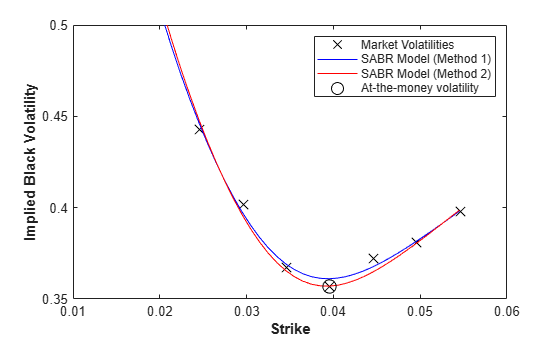# Calibrate SABR Model Using Analytic Pricer

This example shows how to use two different methods to calibrate a SABR stochastic volatility model from market implied Black volatilities. Both approaches use the `SABR` analytic pricer.

### Load Market Implied Black Volatility Data

This example sets up hypothetical market implied Black volatilities for European swaptions over a range of strikes before calibration. The swaptions expire in three years from the `Settle` date and have five-year swaps as the underlying instrument. The rates are expressed in decimals. (Changing the units affects the numerical value and interpretation of the `Alpha` parameter in the `SABR` model.)

Load the market implied Black volatility data for swaptions expiring in three years.

```Settle = datetime(2013, 7, 10); ZeroDates = Settle + [calmonths([1 2 3 6 9]) calyears([1 2 3 4 5 6 7 8 9 10 12])]'; ZeroRates = [0.25 0.3 0.33 0.42 0.8 0.9 1.1 1.2 ... 1.8 2.2 2.4 2.71 2.95 3.02 3.24 3.58]'/100; Compounding = 1; ZeroCurve = ratecurve("zero",Settle,ZeroDates,ZeroRates,'Compounding',Compounding); ExerciseDate = datetime(2016, 7, 10); MarketStrikes = [2.46 2.96 3.46 3.96 4.46 4.96 5.46]'/100; MarketVolatilities = [44.3 40.2 36.7 35.7 37.2 38.1 39.8]'/100;```

At the time of `Settle`, define the underlying forward rate and the at-the-money volatility.

`CurrentForwardValue = MarketStrikes(4)`
```CurrentForwardValue = 0.0396 ```
`ATMVolatility = MarketVolatilities(4)`
```ATMVolatility = 0.3570 ```

### Method 1: Calibrate `Alpha`, `Rho`, and `Nu` Directly

You can calibrate the `Alpha`, `Rho`, and `Nu` model parameters directly. Set the value of `Beta` either by fitting historical market volatility data or by choosing a value appropriate for the market . For this example, use the value `0.5`.

```% Define the predetermined Beta. Beta1 = 0.5;```

After fixing the value of $\beta$ (`Beta`), fit the parameters $\alpha$ (`Alpha`), $\rho$ (`Rho`), and $\nu$ (`Nu`) directly. The Optimization Toolbox™ function `lsqnonlin` generates the parameter values that minimize the squared error between the market volatilities and the volatilities computed by the `SABR` analytic pricer.

```% Calibrate Alpha, Rho, and Nu. objFun = @(X) MarketVolatilities - ... volatilities(finpricer("Analytic", 'Model', ... finmodel("SABR", 'Alpha', X(1), 'Beta', Beta1, 'Rho', X(2), 'Nu', X(3)), ... 'DiscountCurve', ZeroCurve), ExerciseDate, CurrentForwardValue, MarketStrikes); X = lsqnonlin(objFun, [0.5 0 0.5], [0 -1 0], [Inf 1 Inf]);```
```Local minimum found. Optimization completed because the size of the gradient is less than the value of the optimality tolerance. ```
```Alpha1 = X(1); Rho1 = X(2); Nu1 = X(3);```

### Method 2: Calibrating Rho and Nu by Implying Alpha from At-The-Money Volatility

You can also use an alternative calibration method. Set the value of $\beta$ (`Beta`) as in the first method.

`Beta2 = 0.5;`

Next you fit the parameters $\rho$ (`Rho`) and $\nu$ (`Nu`) directly while $\alpha$ (`Alpha`) is implied from the market at-the-money volatility. Models calibrated using this method produce at-the-money volatilities that are equal to market quotes. This approach is widely used in swaptions, where at-the-money volatilities are quoted most frequently and are important to match. To imply $\alpha$ (`Alpha`) from market at-the-money volatility (${\sigma }_{ATM}$), the following cubic polynomial is solved for $\alpha$ (`Alpha`), and the smallest positive real root is selected .

`$\frac{\left(1-\beta {\right)}^{2}T}{24{F}^{\left(2-2\beta \right)}}{\alpha }^{3}+\frac{\rho \beta \nu T}{4{F}^{\left(1-\beta \right)}}{\alpha }^{2}+\left(1+\frac{2-3{\rho }^{2}}{24}{\nu }^{2}T\right)\alpha -{\sigma }_{ATM}{F}^{\left(1-\beta \right)}=0$`

Here:

$F$ is the current forward value

$T$ is the year fraction to maturity.

Fit the parameters by defining an anonymous function.

```% Year fraction from Settle to option maturity. T = yearfrac(Settle, ExerciseDate, 1); % This function solves the SABR at-the-money volatility equation as a % polynomial of Alpha. alpharoots = @(Rho,Nu) roots([... (1 - Beta2)^2*T/24/CurrentForwardValue^(2 - 2*Beta2) ... Rho*Beta2*Nu*T/4/CurrentForwardValue^(1 - Beta2) ... (1 + (2 - 3*Rho^2)*Nu^2*T/24) ... -ATMVolatility*CurrentForwardValue^(1 - Beta2)]); % This function converts at-the-money volatility into Alpha by picking the % smallest positive real root. atmVol2SabrAlpha = @(Rho,Nu) min(real(arrayfun(@(x) ... x*(x>0) + realmax*(x<0 || abs(imag(x))>1e-6), alpharoots(Rho,Nu))));```

The function `atmVol2SabrAlpha` converts at-the-money volatility into $\alpha$ (`Alpha`) for a given set of $\rho$ (`Rho`) and $\nu$ (`Nu`). This function is then used in the objective function to fit the parameters $\rho$ (`Rho`) and $\nu$ (`Nu`).

```% Calibrate Rho and Nu (while converting at-the-money volatility into Alpha % using atmVol2SabrAlpha). objFun = @(X) MarketVolatilities - ... volatilities(finpricer("Analytic", 'Model', finmodel("SABR", ... 'Alpha', atmVol2SabrAlpha(X(1), X(2)), 'Beta', Beta2, 'Rho', X(1), 'Nu', X(2)), ... 'DiscountCurve', ZeroCurve), ExerciseDate, CurrentForwardValue, MarketStrikes); X = lsqnonlin(objFun, [0 0.5], [-1 0], [1 Inf]);```
```Local minimum found. Optimization completed because the size of the gradient is less than the value of the optimality tolerance. ```
```Rho2 = X(1); Nu2 = X(2);```

The calibrated parameter $\alpha$ (`Alpha`) is computed using the calibrated parameters $\rho$ (`Rho`) and $\nu$ (`Nu`).

```% Obtain final Alpha from at-the-money volatility using calibrated % parameters. Alpha2 = atmVol2SabrAlpha(Rho2, Nu2); % Display calibrated parameters. C = {Alpha1 Beta1 Rho1 Nu1;Alpha2 Beta2 Rho2 Nu2}; CalibratedPrameters = cell2table(C,... 'VariableNames',{'Alpha' 'Beta' 'Rho' 'Nu'},... 'RowNames',{'Method 1';'Method 2'})```
```CalibratedPrameters=2×4 table Alpha Beta Rho Nu ________ ____ _______ _______ Method 1 0.060203 0.5 0.19131 0.85327 Method 2 0.058851 0.5 0.18901 0.88627 ```

### Use Calibrated Models

Use the calibrated models to compute new volatilities at any strike value.

Compute volatilities for models calibrated using Method 1 and Method 2 and plot the results.

```PlottingStrikes = (1.75:0.1:5.50)'/100; % Compute volatilities for model calibrated by Method 1. SABR_Model_Method_1 = finmodel("SABR", ... 'Alpha', Alpha1, 'Beta', Beta1, 'Rho', Rho1, 'Nu', Nu1); ComputedVols1 = volatilities(finpricer("Analytic", ... 'Model', SABR_Model_Method_1, 'DiscountCurve', ZeroCurve), ... ExerciseDate, CurrentForwardValue, PlottingStrikes); % Compute volatilities for model calibrated by Method 2. SABR_Model_Method_2 = finmodel("SABR", ... 'Alpha', Alpha2, 'Beta', Beta2, 'Rho', Rho2, 'Nu', Nu2); ComputedVols2 = volatilities(finpricer("Analytic", ... 'Model', SABR_Model_Method_2, 'DiscountCurve', ZeroCurve), ... ExerciseDate, CurrentForwardValue, PlottingStrikes); figure; plot(MarketStrikes,MarketVolatilities,'xk',... PlottingStrikes,ComputedVols1,'b', ... PlottingStrikes,ComputedVols2,'r', ... CurrentForwardValue,ATMVolatility,'ok',... 'MarkerSize',10); xlim([0.01 0.06]); ylim([0.35 0.5]); xlabel('Strike', 'FontWeight', 'bold'); ylabel('Implied Black Volatility', 'FontWeight', 'bold'); legend('Market Volatilities', 'SABR Model (Method 1)',... 'SABR Model (Method 2)', 'At-the-money volatility');```The model calibrated using Method 2 reproduces the market at-the-money volatility (marked with a circle) exactly.

### References

 Hagan, Patrick S., Deep Kumar, Andrew S. Lesniewski, and Diana E. Woodward. "Managing Smile Risk." Wilmott Magazine. (January 2002): 84–108.

 West, Graeme. "Calibration of the SABR Model in Illiquid Markets." Applied Mathematical Finance. 12, no. 4 (December 2005): 371–385.